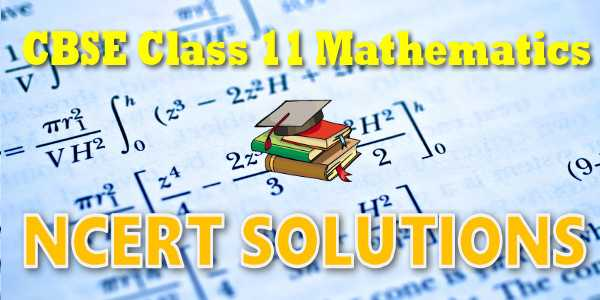# NCERT solutions for class 11 Mathematics## NEET-UG 2021

10,000+ Daily Practice Questions

(only at ₹ 299/- in myCBSEguide App)

## NCERT solutions for class 11 Mathematics## myCBSEguide App

Complete Guide for CBSE Students

NCERT Solutions, NCERT Exemplars, Revison Notes, Free Videos, CBSE Papers, MCQ Tests & more.## NCERT Solutions Chapter 16: Probability

In earlier classes, we studied about the concept of probability as a measure of uncertainty of various phenomenon. We have obained the probability of getting an even number in throwing a die as {tex}\frac{3}{6}{/tex} i.e., {tex}\frac{1}{2}{/tex}. Here the total possible outcomes are 1, 2, 3, 4, 5 and 6 (six in number). The outcomes in favour of the event of 'getting an even number' are 2, 4, 6 (i.e., three in number). In general, to obtain the probability of an event, we find the ratio of the number of outcomes favourable to the event, to the total number of equally likely outcomes. This theory of probability is known as classical theory of probability.

## NCERT Solutions for Class 11th Mathematics

NCERT Solutions Class 11 Mathematics PDF (Download) Free from myCBSEguide app and myCBSEguide website. Ncert solution class 11 Mathematics includes text book solutions. NCERT Solutions for CBSE Class 11 maths have total 16 chapters. Class 11 Mathematics ncert Solutions in pdf for free Download are given in this website. Ncert Mathematics class 11 solutions PDF and Mathematics ncert class 11 PDF solutions with latest modifications and as per the latest CBSE syllabus are only available in myCBSEguide.

## NCERT Solutions for Class 11## myCBSEguide

Trusted by 1 Crore+ Students

#### CBSE Test Generator

Create papers in minutes

Print with your name & Logo

3 Lakhs+ Questions

Solutions Included

Based on CBSE Blueprint

Best fit for Schools & Tutors

#### Work from Home

• Work from home with us
• Create questions or review them from home

No software required, no contract to sign. Simply apply as teacher, take eligibility test and start working with us. Required desktop or laptop with internet connection